# CSE 202 Algorithms Greedy Algorithms 103102 CSE 202

• Slides: 13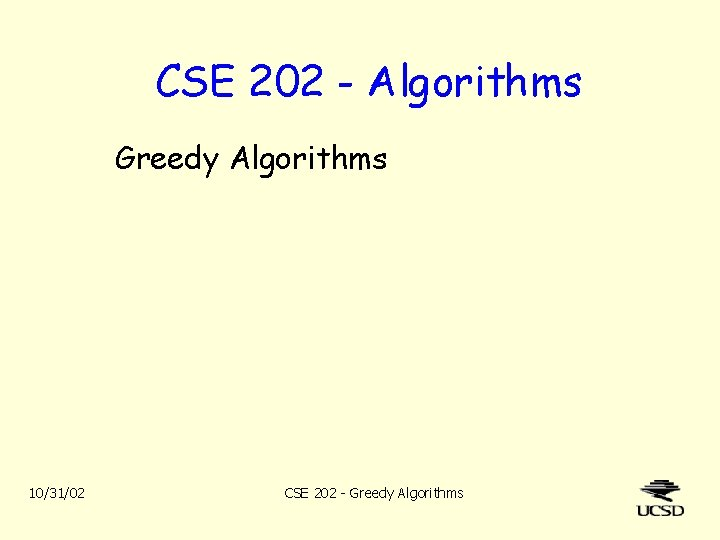CSE 202 - Algorithms Greedy Algorithms 10/31/02 CSE 202 - Greedy AlgorithmsGreedy Algorithms Optimization problem: find the best way to do something. – E. g. match up two strings (LCS problem). Search techniques look at many possible solutions. – E. g. dynamic programming or backtrack search. A greedy algorithm – Makes choices along the way that seem the best. – Sticks with those choices. For some problems, greedy approach always gets optimum. For others, greedy finds good, but not always best. – If so, it’s called a greedy heuristic. or approximation. For still others, greedy approach can do very poorly. 2 CSE 202 - Greedy AlgorithmsThe problem of giving change Vending machine has huge supply of quarters, dimes and nickels. Customer needs N cents change (N is multiple of 5). Machine wants to give out few coins as possible. Greedy approach: while (N > 0) { give largest denomination coin N; reduce N by value of that coin; } Does this return the fewest number of coins? Aside: Using division, it could make decisions faster. 3 CSE 202 - Greedy AlgorithmsMore on giving change Thm: Greedy algorithm always gives minimal # of coins. Proof: – Optimum has 2 dimes. • Quarter and nickel better than 3 dimes. – Optimum has 1 nickel • Dime better than 2 nickels. – Optimum doesn’t have 2 dimes + 1 nickel • It would use quarter instead. – So optimum & greedy have at most \$0. 20 in non-quarters. • That is, they give the same number of quarters. – Optimum & greedy give same on remaining \$0. 20 too. • Obviously. 4 CSE 202 - Greedy AlgorithmsMore on giving change Suppose we run out of nickels, put pennies in instead. – Does greedy approach still give minimum number of coins? Formally, the Coin Change problem is: Given k denominations d 1, d 2, . . . , dk and given N, find a way of writing N = i 1 d 1 + i 2 d 2 +. . . + ik dk such that i 1 + i 2 +. . . + ik is minimized. “Size” of problem is k. Is the greedy algorithm always a good heuristic? That is, is there exists a constant c s. t. for all instances of Coin Change, the greedy algorithm gives at most c times the optimum number of coins? How do we solve Coin Change exactly? 5 CSE 202 - Greedy AlgorithmsCoin Change by Dynamic Programming Let C(N) = min # of coins needed to give N cents. Detail: If N < 0, define C(N) = Optimal substructure: If you remove 1 coin, you must have minimum solution to smaller problem. So C(N) = 1 + min { C(N-5), C(N-10), C(N-25) } 0 5 10 15 20 25 30 35 40 45 50 55 60 65 70 0 1 6 1 1 CSE 202 - Greedy Algorithms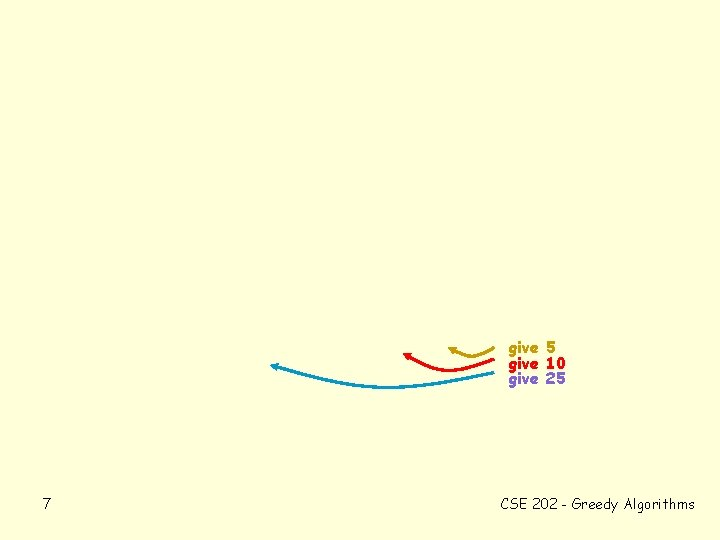give 5 give 10 give 25 7 CSE 202 - Greedy Algorithms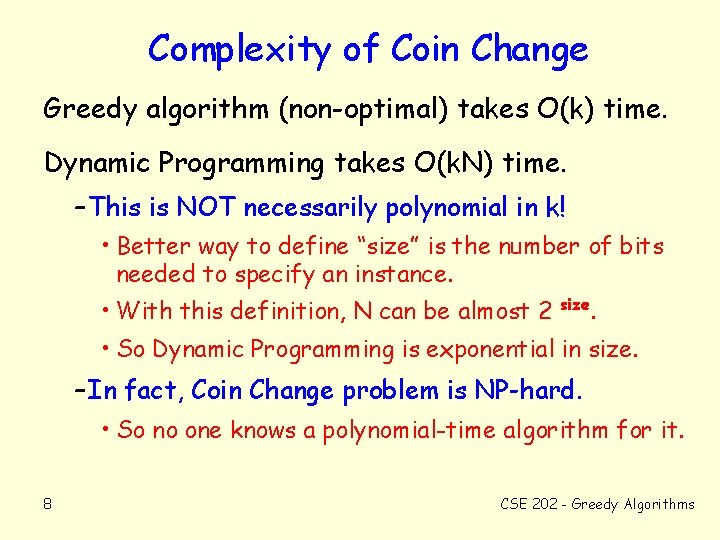Complexity of Coin Change Greedy algorithm (non-optimal) takes O(k) time. Dynamic Programming takes O(k. N) time. – This is NOT necessarily polynomial in k! • Better way to define “size” is the number of bits needed to specify an instance. • With this definition, N can be almost 2 size . • So Dynamic Programming is exponential in size. – In fact, Coin Change problem is NP-hard. • So no one knows a polynomial-time algorithm for it. 8 CSE 202 - Greedy AlgorithmsLinear Partition Problem Given a list of positive integers, s 1, s 2, . . . , s. N, and a bound B, find smallest number of contiguous sublists s. t. each sum of each sublist B. I. e. : find partition points 0 = p 0, p 1, p 2, . . . , pk = N such that for j = 0, 1, . . . , k-1, pj+1 si B Greedy algorithm: i=pj+1 Choose p 1 as large as possible. Then choose p 2 as large as possible. Etc. 9 CSE 202 - Greedy Algorithms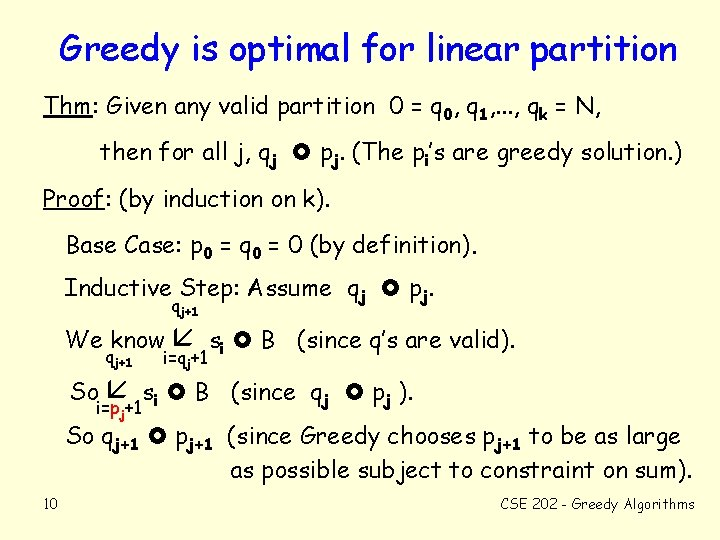Greedy is optimal for linear partition Thm: Given any valid partition 0 = q 0, q 1, . . . , qk = N, then for all j, qj pj. (The pi’s are greedy solution. ) Proof: (by induction on k). Base Case: p 0 = q 0 = 0 (by definition). Inductive Step: Assume qj pj. qj+1 We know qj+1 So si B (since q’s are valid). i=qj+1 si B (since qj pj ). i=pj+1 So qj+1 pj+1 (since Greedy chooses pj+1 to be as large as possible subject to constraint on sum). 10 CSE 202 - Greedy Algorithms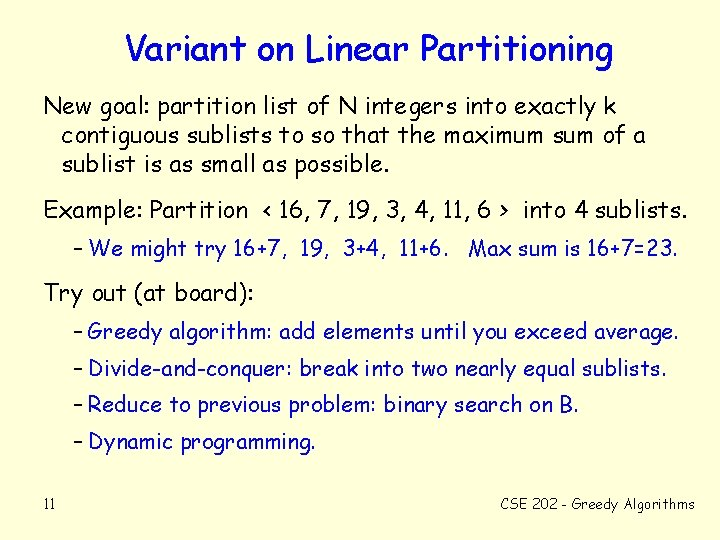Variant on Linear Partitioning New goal: partition list of N integers into exactly k contiguous sublists to so that the maximum sum of a sublist is as small as possible. Example: Partition < 16, 7, 19, 3, 4, 11, 6 > into 4 sublists. – We might try 16+7, 19, 3+4, 11+6. Max sum is 16+7=23. Try out (at board): – Greedy algorithm: add elements until you exceed average. – Divide-and-conquer: break into two nearly equal sublists. – Reduce to previous problem: binary search on B. – Dynamic programming. 11 CSE 202 - Greedy Algorithms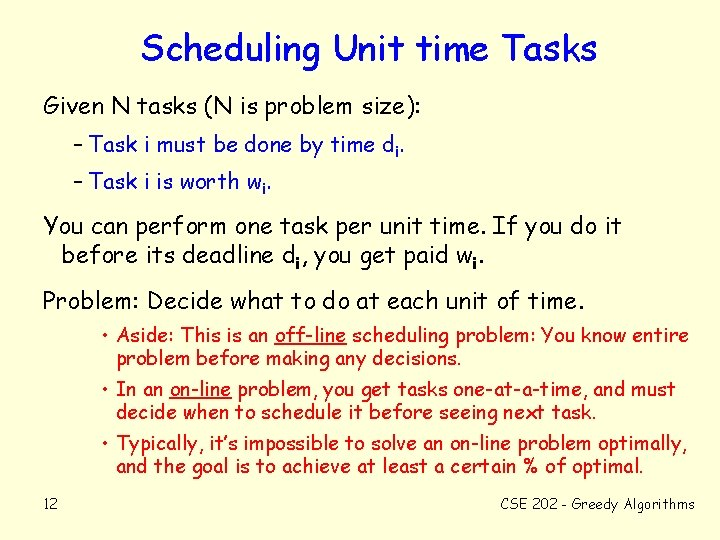Scheduling Unit time Tasks Given N tasks (N is problem size): – Task i must be done by time di. – Task i is worth wi. You can perform one task per unit time. If you do it before its deadline di, you get paid wi. Problem: Decide what to do at each unit of time. • Aside: This is an off-line scheduling problem: You know entire problem before making any decisions. • In an on-line problem, you get tasks one-at-a-time, and must decide when to schedule it before seeing next task. • Typically, it’s impossible to solve an on-line problem optimally, and the goal is to achieve at least a certain % of optimal. 12 CSE 202 - Greedy AlgorithmsGlossary (in case symbols are weird) subset for all element of infinity there exists big theta big omega summation >= <= about equal not equal natural numbers(N) reals(R) 13 rationals(Q) integers(Z) CSE 202 - Greedy Algorithms